Call Choice Point Psychological today: 587-362-0640

In order to examine predictors of PTG subscales and to test the Hypothesis 5 (Different factors will predict each of the five domains of PTG) of the present, hierarchical regression analyses were conducted separately for each subscale of PTG. These subscales are new possibilities, spiritual change, relating to others, personal strength, and appreciation of life.

The results of regression analysis conducted for new possibilities subscale, revealed that control variables explained 5% of the variance. Only being passenger in the accident (β = .18, t = 2.68, p < .01) was found to be a significant predictor of new possibilities.

From personality variables, only agreeableness (β = 28, t = 4.02, p < .001) was a significant predictor of new possibilities and the inclusion of personality variables to the equation increased the explained variance to 12% (R2 change = .07, F change (1, 219) = 16.13, p < .001).

Furthermore, with the event related variables in the equation, the explained variance reached 28% (R2 change = .16, F change (1, 218) = 49.33, p < .001). Only perceived severity of the event was positively associated with new possibilities (β = .45, t = 7.02, p < .001).

From coping and rumination variables, the entrance of deliberate rumination into the equation increased the explained variance to 44% (R2 change = .16, F change (1, 217) = 60.87, p < .001), similarly, with the inclusion of the problem solving coping to the equation the explained variance was increased to 49% (R2 change = .05, F change (1, 216) = 21.03, p < .001). For this last step, significant associates of new possibilities were deliberate rumination (β = .48, t = 7.80, p < .001), and problem solving coping (β = .26, t = 7.59, p < .001).

With all the variables in the equation, gender (t = 2.31, p < .05), agreeableness (t = 2.10, p < .05), perceived severity of the event (t = 3.27, p < .01), deliberate rumination (t = 6.05, p < .001), and problem solving coping (t = 4.59, p < .001) were found to be significant predictors of new possibilities (See Table 16).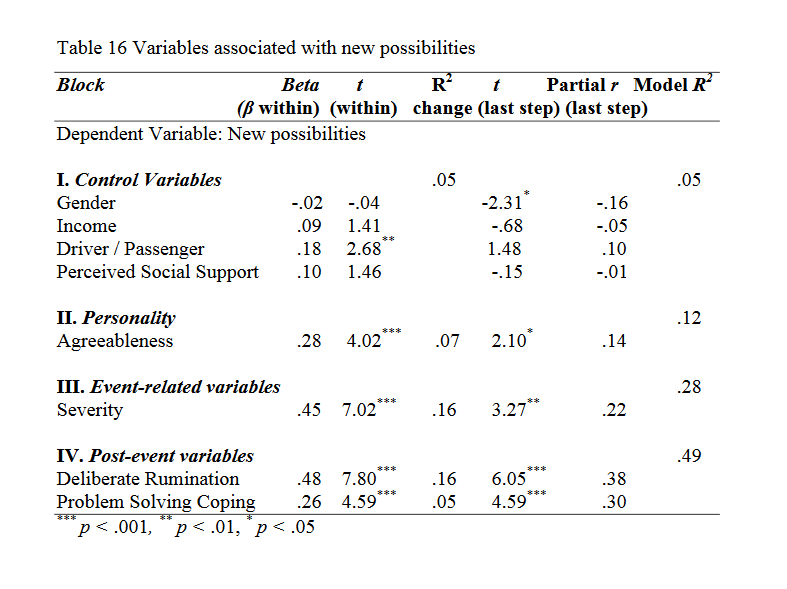As can be seen from Table 17, the results of regression analysis conducted for spiritual change, showed that control variables explained 4% of the variance. From control variables, only being passenger was associated with spiritual change (β = .16, t = 2.39, p < .05).
For spiritual change, none of the personality variables was found to be significant predictor, whereas, with the entrance of event related variables the explained variance reached 22% (R2 change = .18, F change (1, 219) = 50.16, p < .001). Perceived severity of the event was a significant predictor relating positively to spiritual change (β = .46, t = 7.08, p < .001).
In the last step, coping and rumination variables were entered into the equation and the explained variance increased to 46%. With the entrance of fatalistic coping into the regression equation, the explained variance reached 39% (R2 change = .17, F change (1, 218) = 59.90, p < .001); moreover, the explained variance was increased to 46% by the inclusion of deliberate rumination to the equation (R2 change = .07, F change (1, 217) = 28.90, p < .001). From these variables fatalistic coping (β = .44, t = 7.74, p < .001), and deliberate rumination (β = .34, t = 5.38, p < .001) were significant predictors of spiritual change.
With all the variables in the equation, perceived severity of the event (t =2.77, p < .01), fatalistic coping (t = 5.89, p < .001), and deliberate rumination (t = 5.38, p < .001) were found to be significant predictors, all relating positively to spiritual change.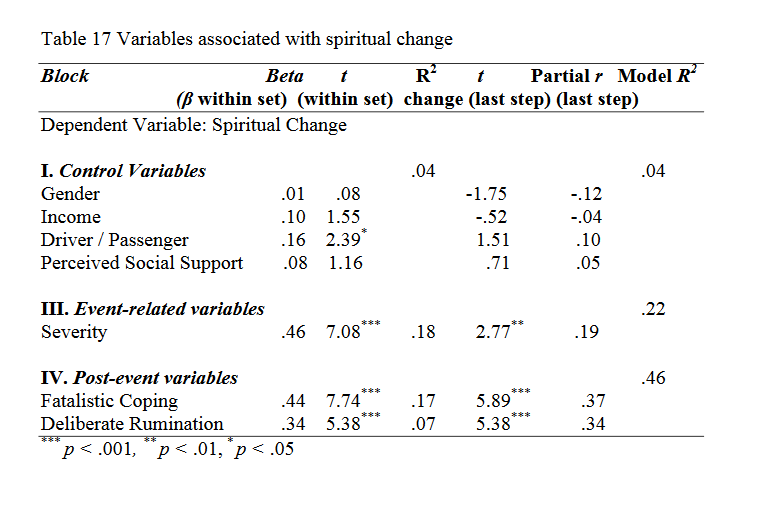The regression analysis conducted for relating to others subscale revealed that control variables explained 8% of the variance. Income (β = .19, t = 2.91, p < .01), and perceived social support (β = .19, t = 2.88, p < .01) were positively associated with relating to others.
With the entrance of personality variables into the equation, only agreeableness (β = .15, t = 2.17, p < .05) was positively associated with relating to others dimension of PTG and it increased the explained variance to 10% (R2 change = .02, F change (1, 219) = 4.71, p < .05).
The inclusion of event related variables to the equation increased the explained variance to 20% (R2 change = .10, F change (1, 218) = 27.77, p < .001). Perceived severity of the event was found to positively predict (β = .35, t = 5.27, p < .001) relating to others subscale of PTG.
Finally, with problem solving coping in the equation, explained variance increased to 37% (R2 change = .17, F change (1, 217) = 60.01, p < .001) and with the entrance of deliberate rumination into the equation the explained variance reached 40% (R2 change = .03, F change (1, 216) = 10.19, p < .01). Both problem solving coping (β = .45, t = 7.75, p < .001), and deliberate rumination (β = .22, t = 3.19, p < .01) were found to be significant predictors of relating to others dimension of PTG.
Therefore, with all variables in the regression equation 40% of the variance was explained. In the last step, perceived severity of the event (t = 2.53, p < .01), problem solving coping (t = 6.31, p < .001), and
deliberate rumination (t = 3.19, p < .01) positively predicted relating to others dimension of PTG (See Table 18).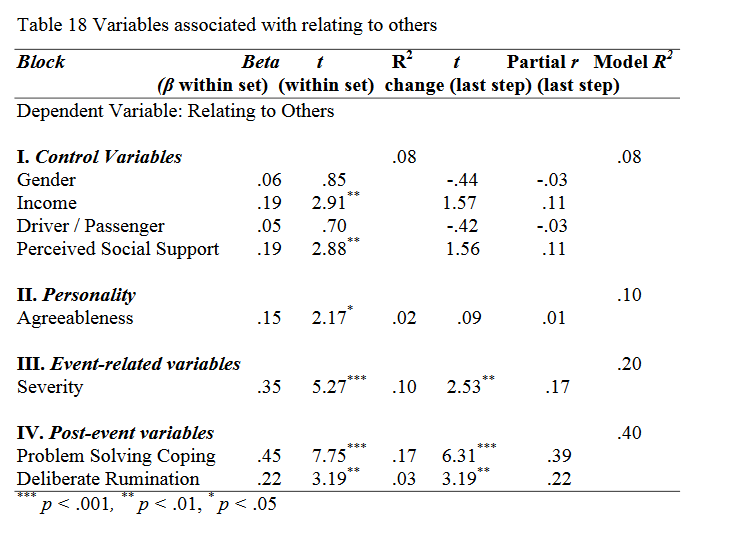According to the results of regression analysis conducted for personal strength subscale, control variables explained 4% of the variance. Only income (β = .14, t = 2.11, p < .05) was positively associated with personal strength.
From personality variables, only agreeableness positively predicted personal strength (β = .21, t = 3.07, p < .01) and it increased the explained variance to 8% (R2 change = .04, F change (1, 219) = 9.41, p < .01).
The addition of event related variables to the equation increased the explained variance to 21% (R2 change = .13, F change (1, 218) = 35.67, p < .001).
In this step, perceived severity of the event was a significant predictor of personal strength (β = .40, t = 5.97, p < .001).
In the last step, from coping and rumination variables, problem solving coping (β = .47, t = 8.28, p < .001), deliberate rumination (β = .27, t = 4.19, p < .001), and fatalistic coping (β = .15, t = 2.21, p < .05) positively predicted personal strength. On the other hand, personal strength was negatively predicted by helplessness coping (β = .17, t = 2.62, p < .01). With the entrance of problem solving coping into the equation, the explained variance of personal strength reached 40% (R2 change = .19, F change (1, 217) = 68.61, p < .001); the inclusion of deliberate rumination to the equation increased the explained variance to 44% (R2 change = .04, F change (1, 216) = 17.52, p < .001). Additionally, with the entrance of fatalistic coping into the regression equation, the explained variance of personal strength reached 45% (R2 change = .01, F change (1, 215) = 4.88, p < .05), and finally with the inclusion of helplessness coping, the explained variance increased to 47% (R2 change = .19, F change (1, 214) = 6.85, p < .01).
With all the variables in the equation, perceived severity of the event (t =2.66, p < .01), problem solving coping (t = 4.73, p < .001), deliberate rumination (t = 4.52, p < .001), and fatalistic coping (t = 2.87, p < .01) remained as the significant predictors, all relating positively to personal strength, whereas helplessness coping (t = 2.62, p < .01) remained negatively predicting personal strength (See Table 19).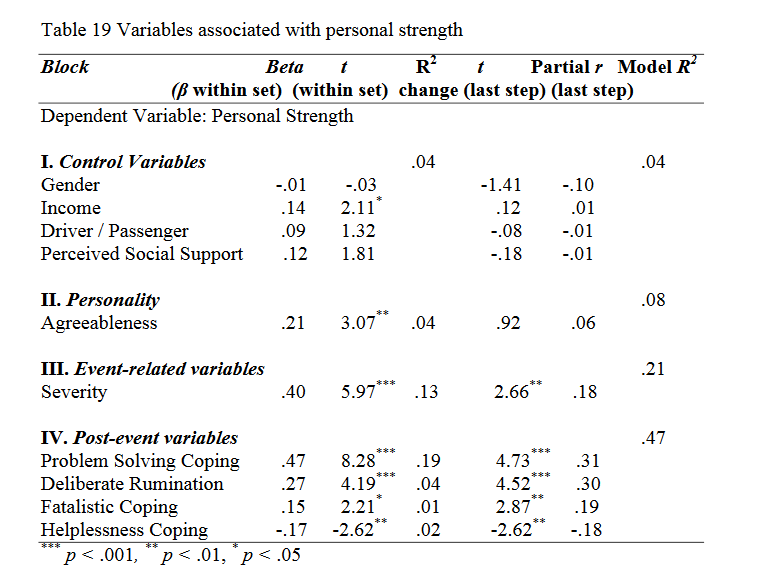The results of regression analysis conducted for appreciation of life subscale showed that 8% of the variance was explained by control variables. Being passenger (β = .21, t = 3.20, p < .01), and perceived social support (β = .16, t = 2.39, p < .05) were positive associates of appreciation of life.
The entrance of personality variables to the equation improved the explained variance to 15% (R2 change = .07, F change (1, 219) = 19.08, p < .001). Only agreeableness (β = .29, t = 4.37, p < .001) was a significant predictor of appreciation of life.
In the following step, perceived severity of the event was positively associated with appreciation of life (β = .42, t = 6.79, p < .001) and its addition to the regression equation improved the explained variance to 30% (R2 change = .15, F change (1, 218) = 46.05, p < .001).
From coping and rumination variables, with the entrance of deliberate rumination into the equation, the explained variance reached 40% (R2 change = .10, F change (1, 217) = 33.85, p < .001), and the inclusion of problem solving coping increased the explained variance of appreciation of life to 43% (R2 change = .03, F change (1, 216) = 11.65, p < .01). These variables namely, deliberate  rumination (β = .37, t = 5.82, p < .001), and problem solving coping (β = .20, t = 3.41, p < .01) positively predicted appreciation of life subscale.
With all the variables in the equation, in the last step, agreeableness (t = 2.80, p < .01), perceived severity of the event (t = 3.61, p < .001), deliberate rumination (t = 4.40, p < .001), and problem solving coping (t = 3.41, p < .01) remained predicting appreciation of life (See Table 20).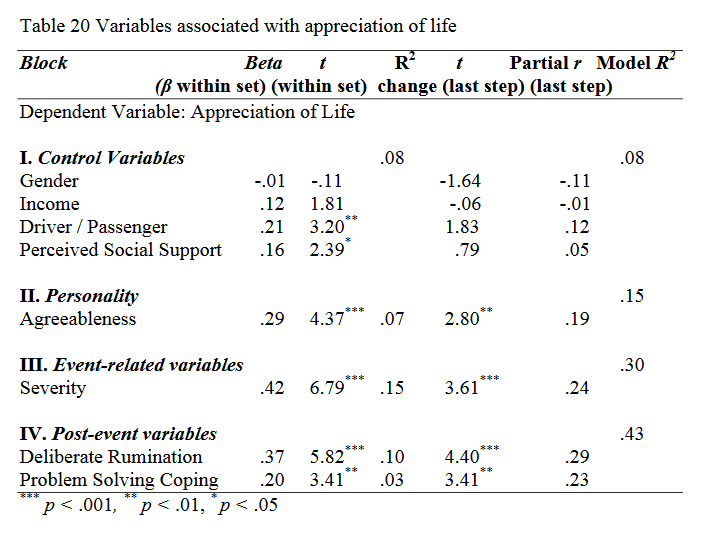Table 21 summarizes the results of the hierarchical regression analyses performed to examine the predictors of PTG and its subscales.